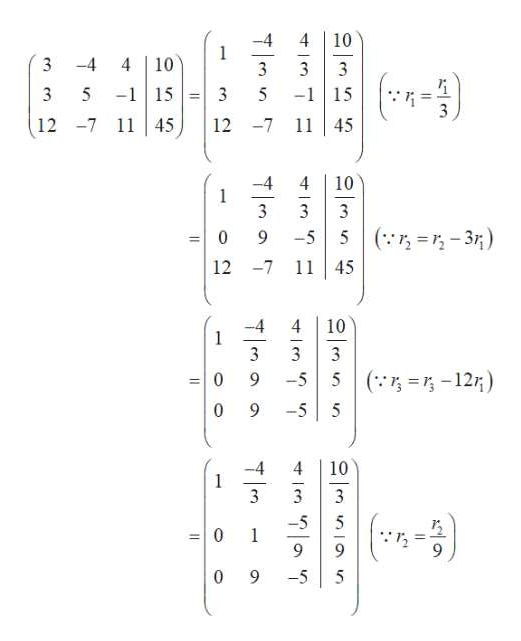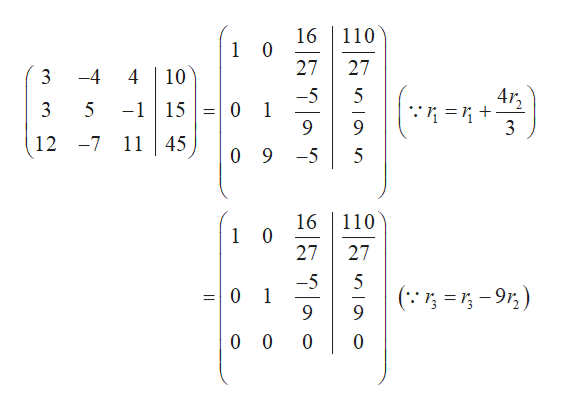# Use the​ Gauss-Jordan method to solve the following system of equations. 3x - 4y + 4z = 103x + 5y - z = 1512x - 7y + 11z =45  Select the correct choice below​ and, if​ necessary, fill in the answer box to complete your choice.  A.The solution is ( ___ , ___ ,___ ) in the order​ x, y, z.​(Simplify your​ answers.) B.There is an infinite number of solutions.The solution is ( ___ , ____, ____ ) where z is any real number.​(Simplify your answers. Use integers or fractions for any numbers in the​ expressions.) C.There is no solution.

Question
14 views
Use the​ Gauss-Jordan method to solve the following system of equations.

3x - 4y + 4z = 10
3x + 5y - z = 15
12x - 7y + 11z =45

Select the correct choice below​ and, if​ necessary, fill in the answer box to complete your choice.

A.
The solution is ( ___ , ___ ,___ ) in the order​ x, y, z.

B.
There is an infinite number of solutions.
The solution is ( ___ , ____, ____ ) where z is any real number.
​(Simplify your answers. Use integers or fractions for any numbers in the​ expressions.)

C.
There is no solution.
check_circle

Step 1

Given system of equations are,

Step 2

By using Gauss-Jordan method solve the system of equations as follows.help_outlineImage Transcriptionclose-4 1 3 4 10 3 -4 10 4 3 3 -1 15 3 -1 15 3 5 3 45 -7 11 12 12 -7 11 45 4 4 10 1 3 3 0 9 ( 3 -5 5 12 -7 11 45 -4 1 3 4 10 3 3 ( 12) -5 5 10 9 -5 -4 1 3 4 10 3 3 -5 1 9 9 0 9 5 -5 fullscreen
Step 3

On further simpli...help_outlineImage Transcriptionclose16 1 0 27 110 27 3 -4 4 10 4r2 0 1 -1 15 3 5 3 1145 12 -7 0 9 -5 5 16 1 0 27 110 27 5 0 1 ( 9 11 0 0 0 fullscreen

### Want to see the full answer?

See Solution

#### Want to see this answer and more?

Solutions are written by subject experts who are available 24/7. Questions are typically answered within 1 hour.*

See Solution
*Response times may vary by subject and question.
Tagged in

### Other